# When using the jacobian determinate to change variables of a double integral, how is it introduced in the first place?

From this page, it discusses the use of polar coordinates in double integrals.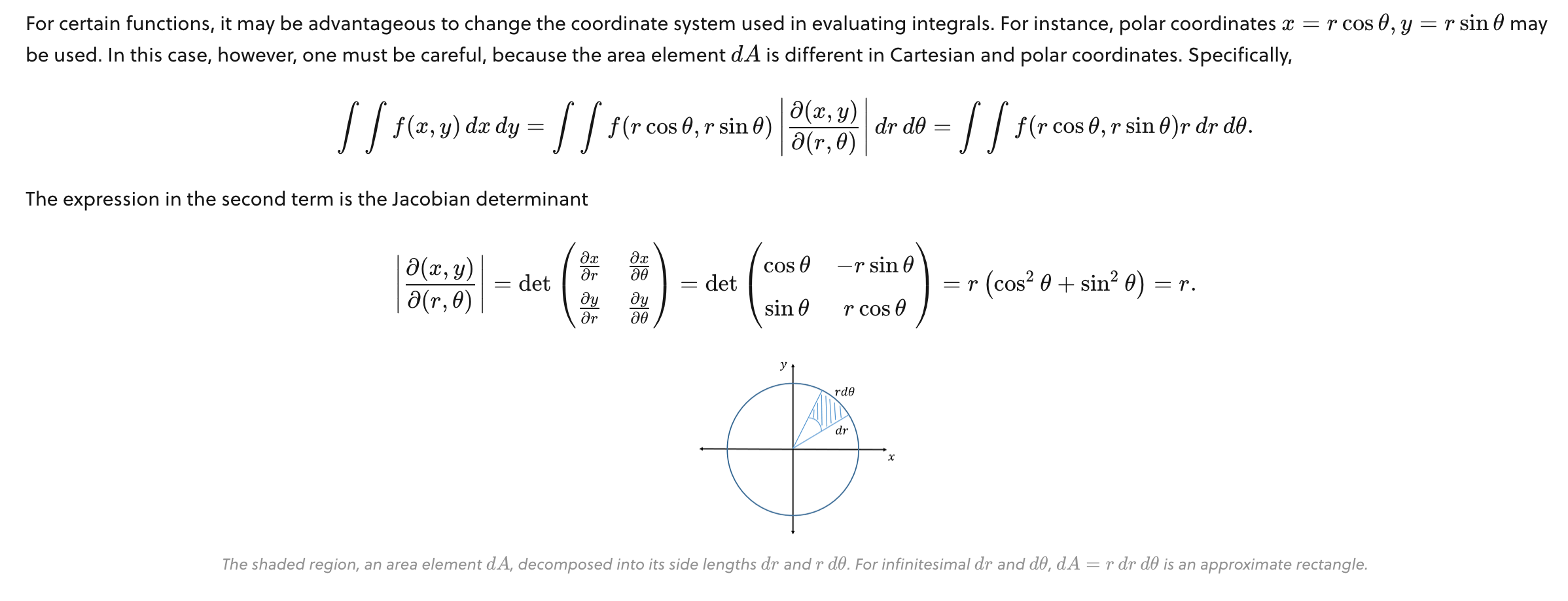In the first line, how and why does $\left|\frac{\partial \left(x,y\right)}{\partial \:\left(r,\theta \right)}\right|$ get introduced in order to change variables? What does this fraction even mean? Im pretty sure its just the absolute value of the partial derivatives with respect to the variables in the brackets. I understand why $f(x,y)$ and $dxdy$ change, but $\left|\frac{\partial \left(x,y\right)}{\partial \:\left(r,\theta \right)}\right|$ pops out seemingly out of nowhere.

My second question is: in the second equation, how does $\left|\frac{\partial \left(x,y\right)}{\partial \:\left(r,\theta \right)}\right|=\begin{pmatrix}\frac{\partial x}{\partial r}&\frac{\partial \:x}{\partial \:\theta }\\ \frac{\partial \:y}{\partial \:r}&\frac{\partial \:y}{\partial \:\theta }\end{pmatrix}$??

Thank youNote by Harry Lam
3 months, 3 weeks ago

This discussion board is a place to discuss our Daily Challenges and the math and science related to those challenges. Explanations are more than just a solution — they should explain the steps and thinking strategies that you used to obtain the solution. Comments should further the discussion of math and science.

When posting on Brilliant:

• Use the emojis to react to an explanation, whether you're congratulating a job well done , or just really confused .
• Ask specific questions about the challenge or the steps in somebody's explanation. Well-posed questions can add a lot to the discussion, but posting "I don't understand!" doesn't help anyone.
• Try to contribute something new to the discussion, whether it is an extension, generalization or other idea related to the challenge.
• Stay on topic — we're all here to learn more about math and science, not to hear about your favorite get-rich-quick scheme or current world events.

MarkdownAppears as
*italics* or _italics_ italics
**bold** or __bold__ bold
- bulleted- list
• bulleted
• list
1. numbered2. list
1. numbered
2. list
Note: you must add a full line of space before and after lists for them to show up correctly
paragraph 1paragraph 2

paragraph 1

paragraph 2

[example link](https://brilliant.org)example link
> This is a quote
This is a quote
    # I indented these lines
# 4 spaces, and now they show
# up as a code block.

print "hello world"
# I indented these lines
# 4 spaces, and now they show
# up as a code block.

print "hello world"
MathAppears as
Remember to wrap math in $$ ... $$ or $ ... $ to ensure proper formatting.
2 \times 3 $2 \times 3$
2^{34} $2^{34}$
a_{i-1} $a_{i-1}$
\frac{2}{3} $\frac{2}{3}$
\sqrt{2} $\sqrt{2}$
\sum_{i=1}^3 $\sum_{i=1}^3$
\sin \theta $\sin \theta$
\boxed{123} $\boxed{123}$

## Comments

Sort by:

Top Newest

My view is very plain and I can answer only one of your questions ( I don’t know anything about double and triple integrals or Jacobian or anything ) but when you change $f(x,y)$ to $f(r \cos θ, r \sin θ)$, the function’s inputs remains the same but changing $\text{d}x \text{d}y$ to $\text{d}r, \text{d}θ$ is not the same and to do this change you need to find out how $\text{d}x \text{d}y$ changes with respect to $\text{d}r, \text{d}θ$, this is why I think the determinant suddenly pops up, why is it that exactly idk, I can only tell that something had to come. @Mark Hennings @Pi Han Goh will surely know this, I am sure they can answer your second question

- 3 months, 3 weeks ago

Log in to reply

Courtesy of James Stewart textbook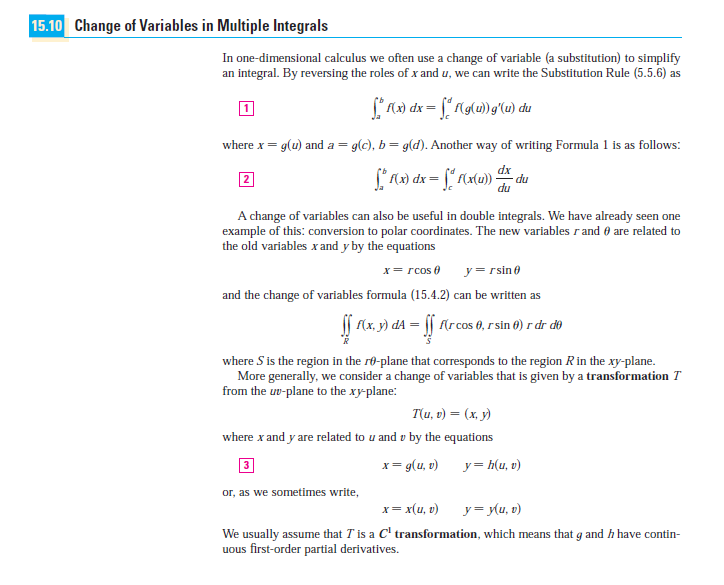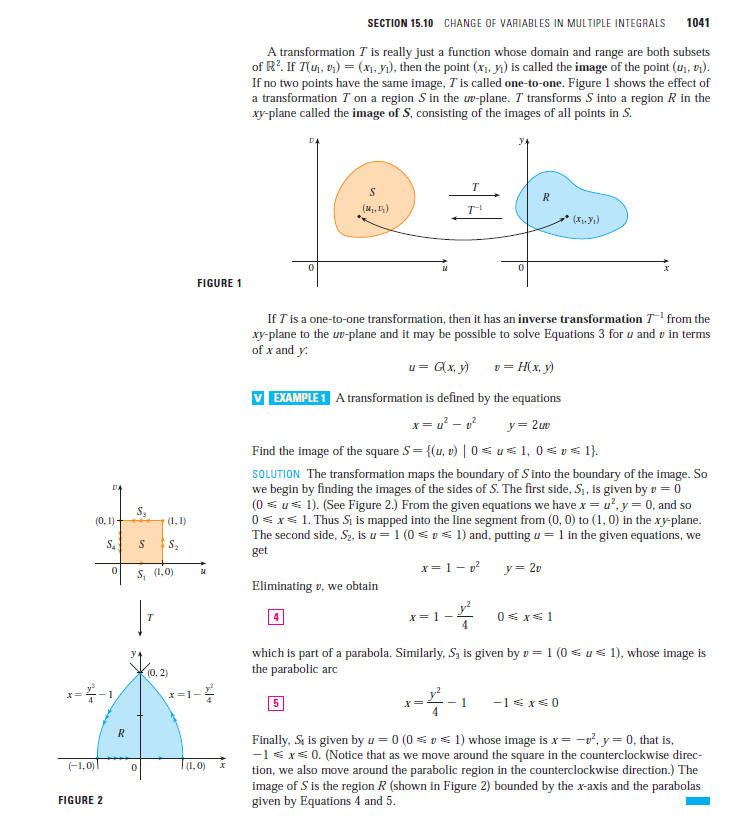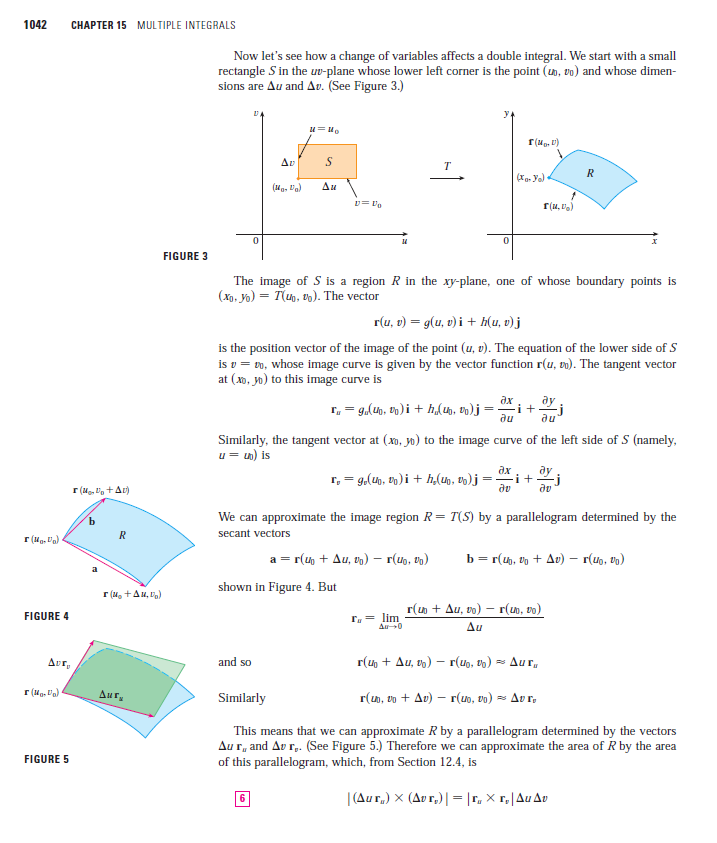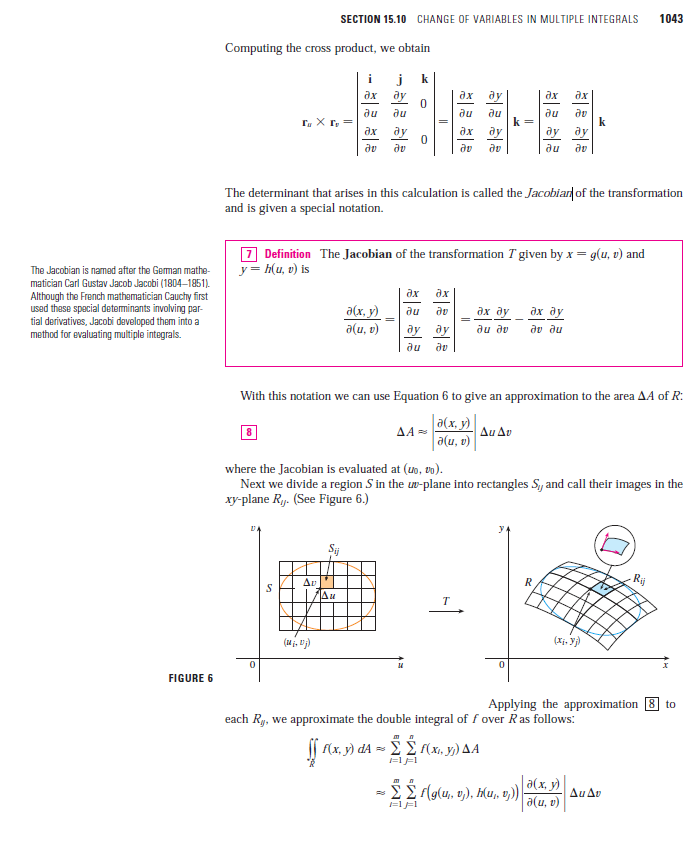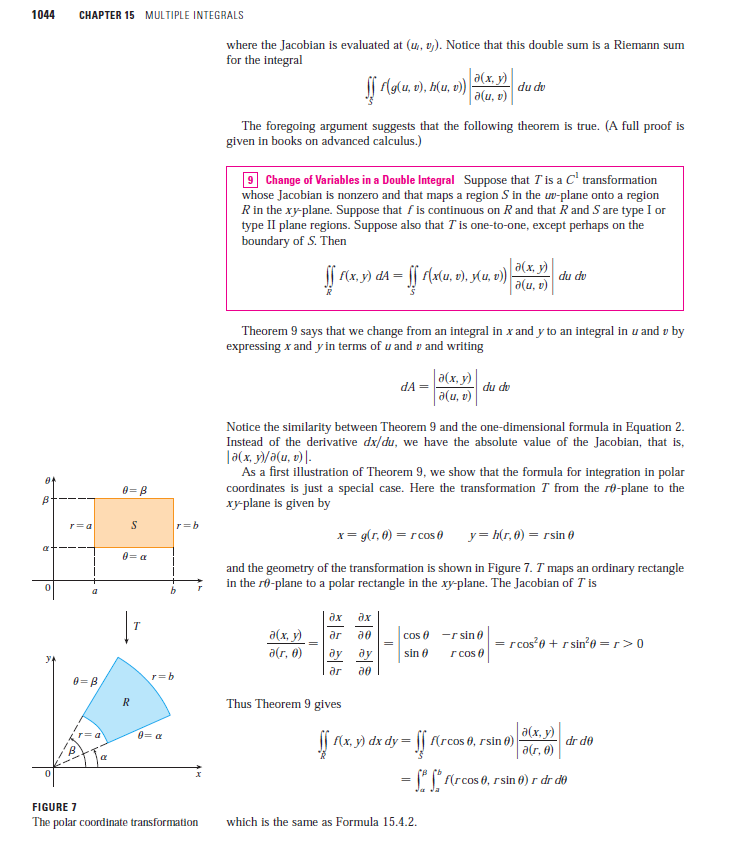- 3 months, 3 weeks ago

Log in to reply

Thanks for this - I have the general gist of things. However, an ELI5 would be very helpful (if possible)

- 3 months, 2 weeks ago

Log in to reply

×

Problem Loading...

Note Loading...

Set Loading...